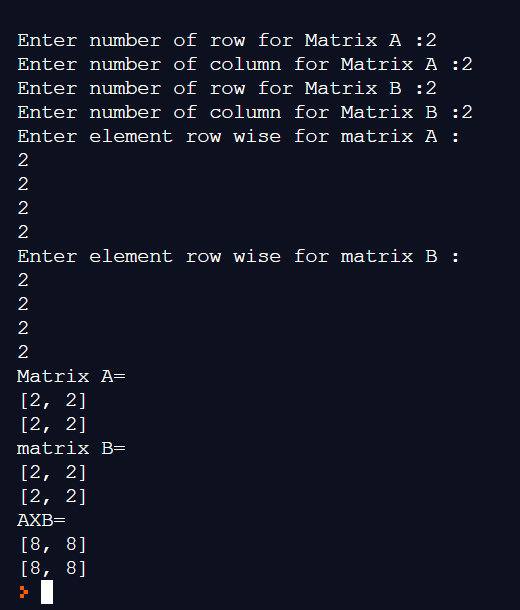# Python 3 Program To Multiply Two Matrices

Here you will learn a simple Python 3 program to multiply two matrices. Before moving ahead, make sure that you have a basic understanding of Python programming concepts such as if-else statements, for loops, lists, dictionaries, etc.

If you are beginning your Python coding journey, then check out these best books to learn Python.

Also Read35 Funny And Best Python Programming Memes.

## Python 3 Program To Multiply Two Matrices

Here is the flow of our Python program.

Step 1: Take input of the number of rows and columns for Matrix A and B.

Step 2: If columns of Matrix A is not equal to rows of matrix B, then the multiplication can’t be done, and the user will see the error message.

Step 3: If columns of matrix A = rows of matrix B, then user’s will be prompted to enter the elements of both the matrices one by one.

Step 4: After the user has specified all the elements, they will see matrix A, matrix B, and the multiplication result.

Now, take a look at the source code and see how all those steps mentioned above are executed.

## Source Code

```r1 = int(input("Enter number of row for Matrix A :"))
c1 = int(input("Enter number of column for Matrix A :"))
r2 = int(input("Enter number of row for Matrix B :"))
c2 = int(input("Enter number of column for Matrix B :"))

if c1 != r2:
print("\nCan't Multiply --> To Multiply two matrix (A x B) ,Column of Matrix A = Row of Matrix B")
else:
print("Enter element row wise for matrix A :")
A = []
for i in range(r1):
arrA = []
for j in range(c1):
arrA.append(int(input()))
A.append(arrA)

print("Enter element row wise for matrix B :")
B = []
for i in range(r2):
arrB = []
for j in range(c2):
arrB.append(int(input()))
B.append(arrB)

print("Matrix A=")
for w in A:
print(w)
print("matrix B=")
for x in B:
print(x)
result = []
for i in range(r1):
arrR = []
for j in range(c2):
arrR.append(0)
result.append(arrR)
for i in range(len(A)):
for j in range(len(B)):
for k in range(len(B)):
result[i][j] = A[i][k] * B[k][j] + result[i][j]

print("AXB=")
for y in result:
print(y)```

## Program output:Enter number of row for Matrix A :2
Enter number of column for Matrix A :2
Enter number of row for Matrix B :2
Enter number of column for Matrix B :2
Enter element row wise for matrix A :
2
2
2
2
Enter element row wise for matrix B :
2
2
2
2
Matrix A=
[2, 2]
[2, 2]
matrix B=
[2, 2]
[2, 2]
AXB=
[8, 8]
[8, 8]

Scroll to Top